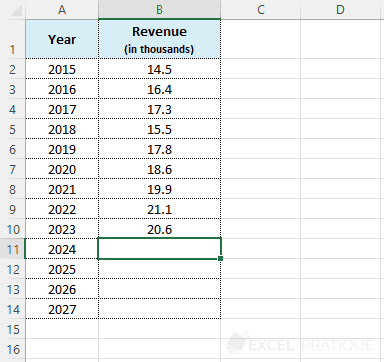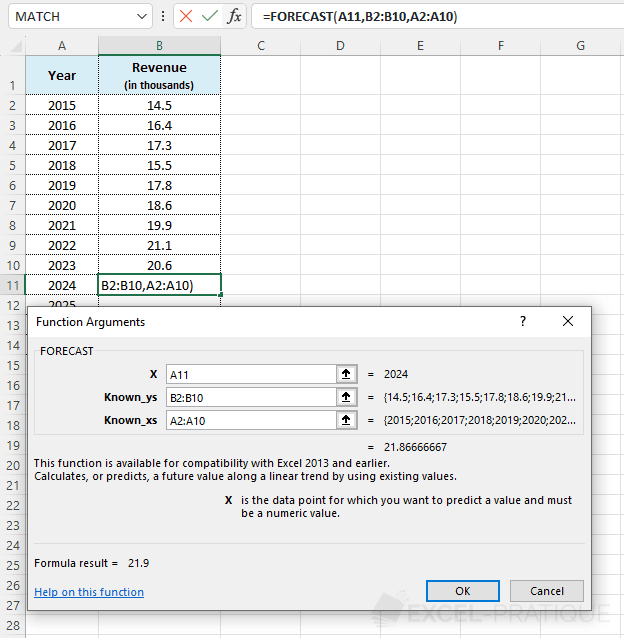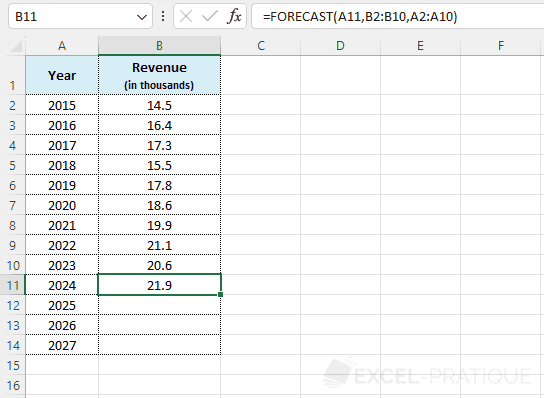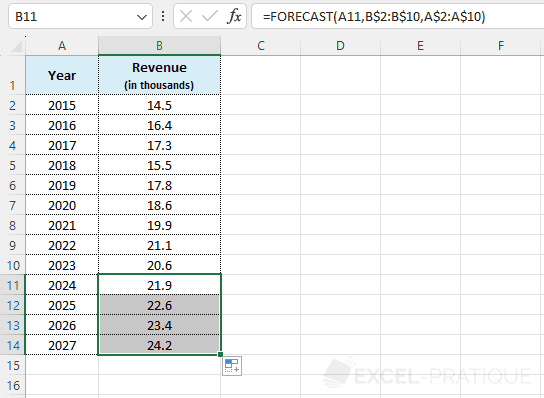# Excel Function: FORECAST

The Excel function FORECAST extrapolates a new data point based on known data.

Usage:

`=FORECAST(X, known_Ys, known_Xs)`

## Example of use

The aim here is to estimate revenue based on the evolution of previous years' revenue:Select the FORECAST function and enter:

• X: the year for which the revenue needs to be estimated
• known_Ys: the range of cells containing the revenue for previous years
• known_Xs: the range of cells containing the years
``=FORECAST(A11,B2:B10,A2:A10)``Click OK.All that remains is to add \$ and drag the formula down: